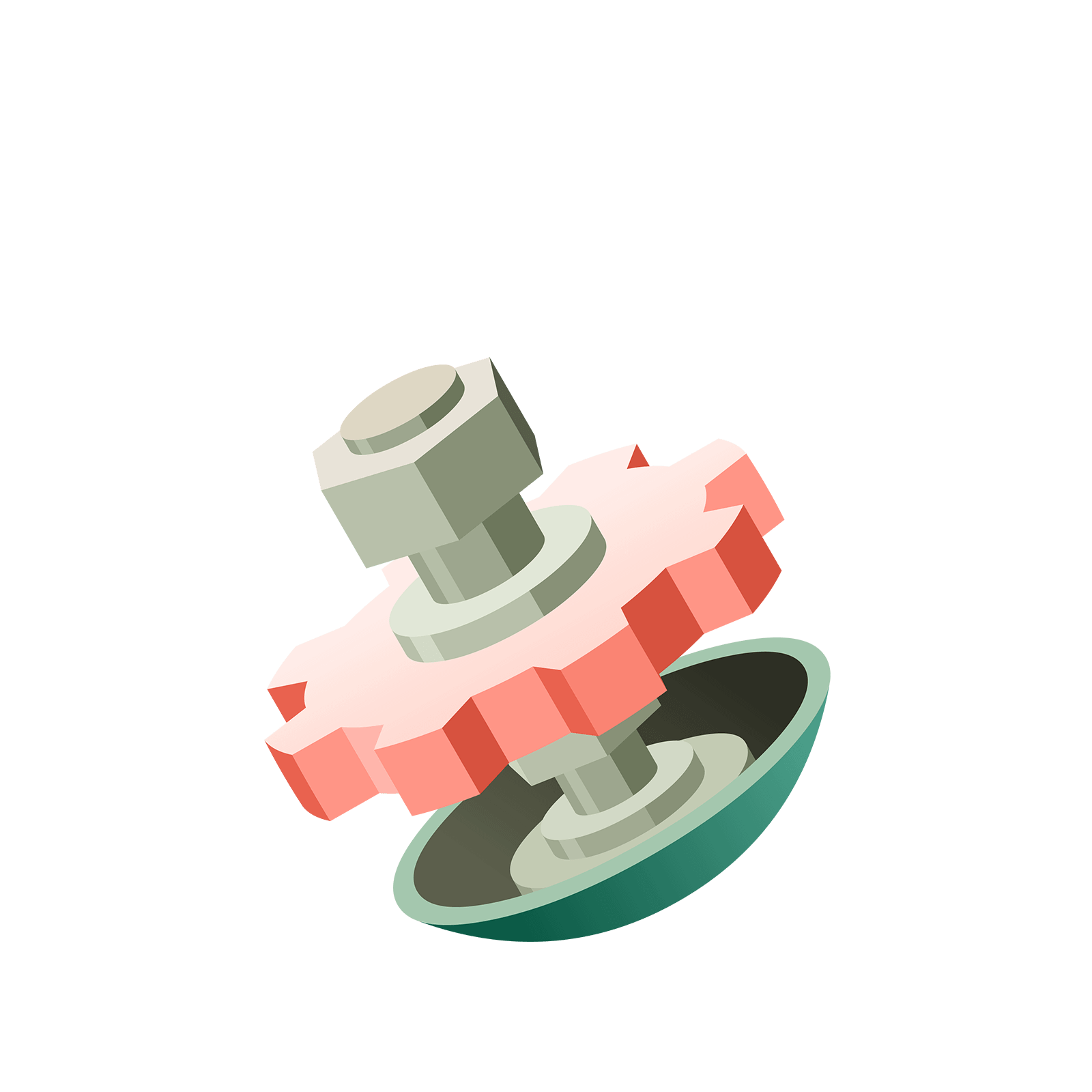06 285 032# Station  3The 1 ← 10 Machine

### Question 1

Here are $273$ dots loaded into a $1 \leftarrow 10$ machine.Use the magic to count the number of explosions that occur from the 1st to the 2nd box.### Question 2

The machine is still unstable. Perform some more explosions.### Question 3

What is the $1 \leftarrow 10$ machine code for the number thirteen?### Question 4

What is the code for $37$ in the $1\leftarrow 10$ machine?### Question 5

What is the $1\leftarrow 10$ machine code for the number $5846$?Something mighty curious is indeed going on with these Exploding Dots machines!
The $1\leftarrow 10$ machine codes are the codes we use in everyday life!
WHAT IS GOING ON?

This is the end of this first island. You can either play with some of the optional stations below or go to the next island!

### Let's Go Wild!